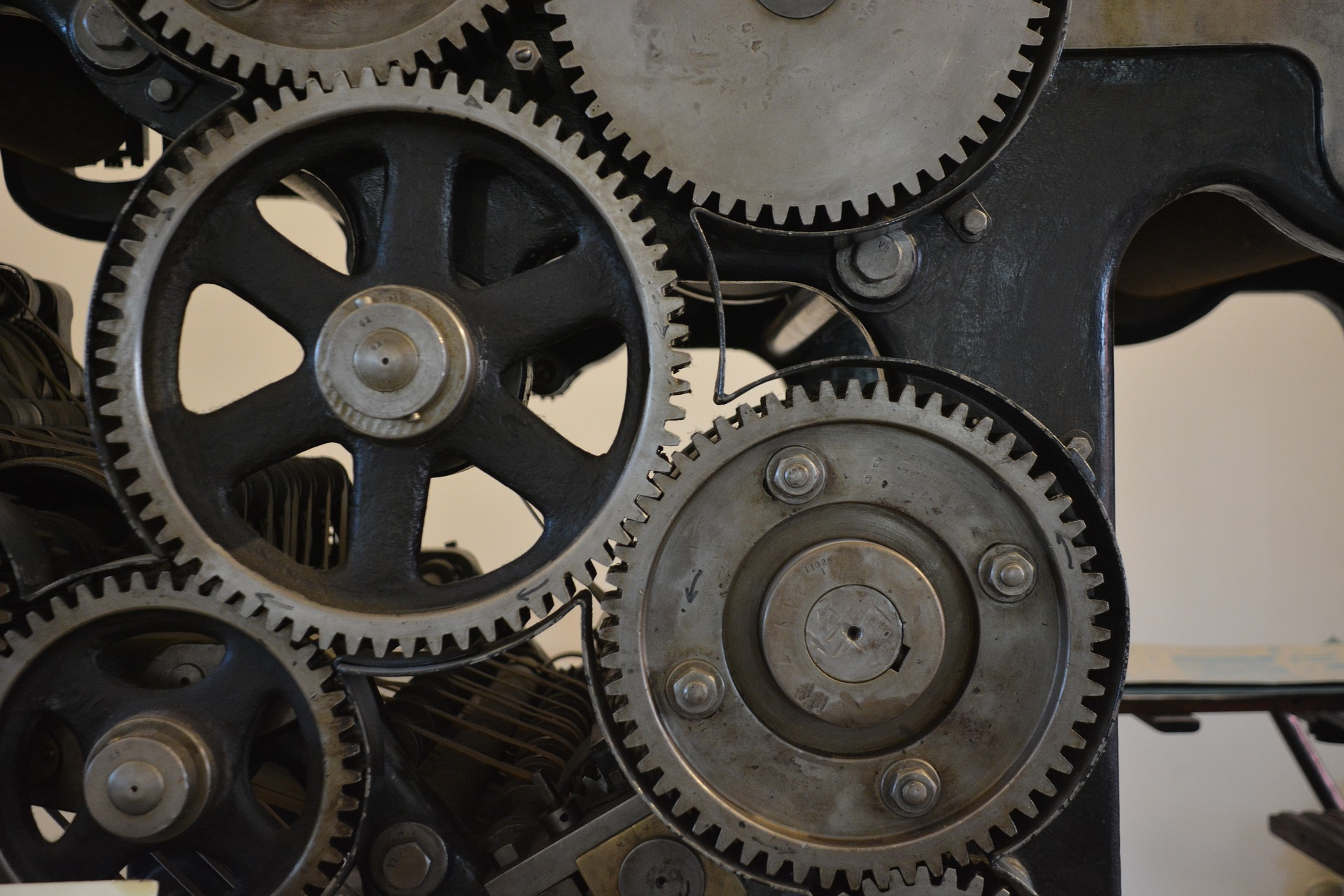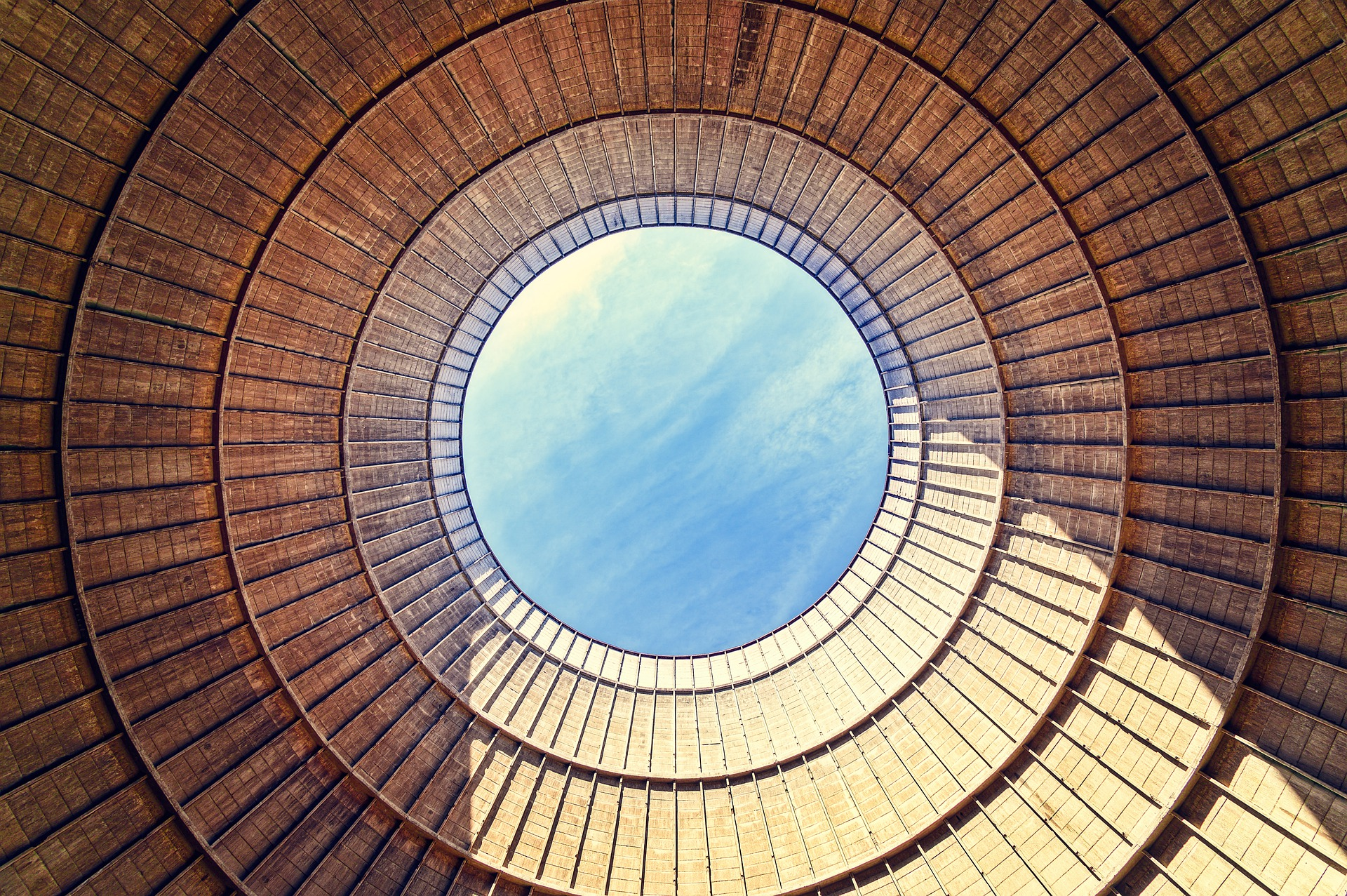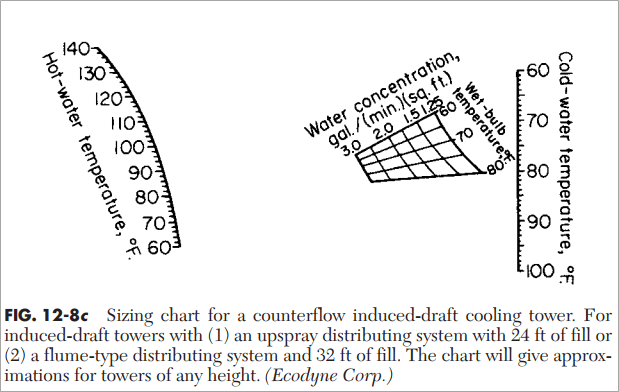## Reciprocating Compressor Power Calculation Part 2 (Three-Stage Compression)

In previous post, I shared how to calculate reciprocating compressor power if number of compression stage is two. In this post, I will use the same problem/example to estimate reciprocating compressor power if number of compression stage is 3. In the end of this posting series, I will show you the difference of each stage and to see how important to determine number of compression stages.

The method used to calculate reciprocating compressor is the same, whether it is two-stage, three-stage, or four-stage. There are several steps which is repetitive. Read More## Reciprocating Compressor Power Calculation Part 1 (Two-Stage Compression)

Many of readers in of this technical blog asked me how to calculate reciprocating compressor power. Long time ago I wrote how to estimate centrifugal compressor power.

In this post I want to share how to calculate reciprocating compressor power. This calculation is used for estimation only. For more accurate results, you should consult a compressor manufacturer.

You will find that the method used in this calculation rely on graphical methods. So, please be patient in using this method.

I used calculation method as stated in GPSA.

Let’s start using example. I used example as used in GPSA. Read More## Comparison of Several Pipeline Flow Equation: Which One is The Most Conservative?

In this post, I want to share to you comparison of several pipeline flow equation. Some of you may already know that there are so many flow equations in pipeline. If you want to understand the details of each equation, I recommend you to check this book. I also use the book as reference in this post.

In general, each flow equation is function of gas flow rate, gas properties, pipeline diameter, pipeline length, and upstream and downstream pressure. There are eleven (11) flow equation described in the book. Read More## I Tried Using Aioflo Pipe Sizing and Flow Calculation

In this post, I want to share with you my experience using Aioflo Pipe Sizing and Flow Calculation. I found this software when searching for Uconeer professional unit conversion program.

Katmar Software developed many engineering software, one of them is Aioflo Pipe Flow Calculator. Aioflo can calculate pipe sizes, flowrate, and pressure drops for liquids and gases. This is a screenshot of the software.## Stage Separation of Gas-Condensate

Stage separation of gas-condensate is a process in which hydrocarbon mixtures are separated into vapor and liquid phases by flashing to low pressure in several steps. Main purposes of stage separation are:

1. To obtain a more stable stock-tank liquid
2. To increase liquid recovery

Figure below shows typical stage separation process.

I want to share to you some terminology that I also just knew about stage separation. A two-stage separation requires one separator and one storage tank, and a three-stage separation requires two separators and one storage tank. I never thought before that the storage tank is always counted as final stage of vapor-liquid separation. Read More## Cost Estimation using Factorial Method

Capital cost estimates for chemical process plants are usually based on purchase cost estimation for major equipment items. The accuracy of this type of estimate will depend on:

• What stage of design has reached
• Time the estimate is made
• Reliability of data available on equipment cost## How to Estimate Pump Efficiency

In this post I want to share to you how to estimate pump efficiency. An equation was developed by Carl Branan, author of the Process Engineer’s Pocket Handbook. Pump efficiency can be estimated by using equation below:Where:

Eff.         = Pump percentage efficiency

G             = Flow (GPM) Read More## How To Estimate Optimum Insulation Thickness

In previous post I shared how to estimate insulation thickness for both flat surface and cylindrical surface. In this post I want to share how to estimate optimum insulation thickness.

The total annual cost of insulation is the sum of the cost of heat energy lost and fixed cost. A plot of cost vs insulation thickness will determine the most economical insulation thickness. A dimensionless factor can be used to calculate insulation thickness, depending on the ratio of insulation thickness to pipe diameter in the following equation:## How To Estimate Insulation Thickness

In this post I want to share how to estimate insulation thickness. Charts in this post show how to estimate insulation thickness for stagnant and turbulent air conditions. Be noted, that the charts are based on neglecting the resistances caused by both the metal and the inside film at the inner wall.

Estimating Insulation Thickness for Flat Surface Read More## Sizing and Horse Power Estimation of Counterflow Induced-Draft Cooling Tower

In this post I want to share to you how to do preliminary sizing and horse power estimation for counterflow induced-draft cooling tower. Calculation in this post parallel or cross-flow cooling tower. Also they do not apply when the approach to cold water temperature is less than 5oF (2.8oC).

Approach temperature is the temperature of the water leaving the cooling tower minus ambient wet bulb temperature.

[Read more: Assessment of Cooling Tower Performance]

To do this, we need this data:

• Hot water temperature
• Cold water temperature
• Wet-bulb temperature
• Water rate

Let’s start sizing the estimation. Let say we have cooling tower with the following process data:

• Hot water temperature = 102oF
• Cold water temperature = 78oF
• Wet-bulb temperature = 70oF
• Water rate = 1000 gallon per minute

Step 1: Find water concentration by using chart

Chart below is used to find water concentration, which is expressed in gallon per minute per square feet (gal/(min.ft2)).When hot water temperature is 102oF and cold water temperature is 78oF, we get water concentration at around 2.8 gal/(min.ft2).

Step 2: Calculate required area of cooling tower

Required area of cooling tower is quantity of water circulated divided by water concentration = 1000/2.8 = 357.14 ft2.

Step 3: Find horsepower per area of cooling tower

Horsepower per area of cooling tower is estimated by using the following chart. Connecting the point representing 100 percent of standard tower performance with the turning point and extending this straight line to the horsepower scale show that it will give around 0.04 hp/ft2 of actual effective tower area. For a tower 357.14 ft2 (see Step 2), 14.6 hp is required to perform the necessary cooling.Step 4: Check if commercial tower size is less than required area

Suppose the actual commercial tower size has an area of only 300 ft2. Within reasonable limit, the shortage of actual area can be compensated by increasing air velocity through the tower. This requires boosting fan horsepower to achieve 110 percent of standard tower performance. From chart in Step 3, we found the fan horse power is 0.057 hp/ft2 of actual area, or 0.057 x 300 = 17.1 hp.

Step 5: Check if commercial tower size is more than required area

On the other hand, if the actual commercial tower size is 370 ft2, the cooling equivalent to 357.14 ft2 of standard tower area can be accomplished with less air and less fan horsepower. From figure in Step 3, the fan horsepower for a tower operating at 90 percent of standard performance is 0.028 hp/ft2 of actual tower area, or 370 x 0.028 = 10.4 hp.

Reference:

Perry’s Chemical Engineers’ Handbook.